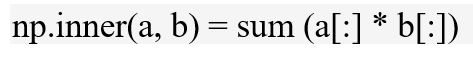Related Articles

# numpy.inner() in python

• Last Updated : 28 Nov, 2018

`numpy.inner(arr1, arr2)`: Computes the inner product of two arrays.```Parameters :
arr1, arr2 : array to be evaluated.

Return:  Inner product of the two arrays.```

Code #1 :

 `# Python Program illustrating ``# numpy.inner() method `` ` `import` `numpy as geek `` ` `# Scalars ``product ``=` `geek.inner(``5``, ``4``) ``print``(``"inner Product of scalar values : "``, product) `` ` `# 1D array ``vector_a ``=` `2` `+` `3j``vector_b ``=` `4` `+` `5j`` ` `product ``=` `geek.inner(vector_a, vector_b) ``print``(``"inner Product : "``, product) `

Output:

```inner Product of scalar values :  20
inner Product :  (-7+22j)
```

Code #2 : As normal matrix multiplication

 `# Python Program illustrating ``# numpy.inner() method `` ` `import` `numpy as geek `` ` `# 1D array ``vector_a ``=` `geek.array([[``1``, ``4``], [``5``, ``6``]]) ``vector_b ``=` `geek.array([[``2``, ``4``], [``5``, ``2``]]) `` ` `product ``=` `geek.inner(vector_a, vector_b) ``print``(``"inner Product : \n"``, product) `` ` `product ``=` `geek.inner(vector_b, vector_a) ``print``(``"\ninner Product : \n"``, product) `

Output:

```inner Product :
[[18 13]
[34 37]]

inner Product :
[[18 34]
[13 37]]
```

Attention geek! Strengthen your foundations with the Python Programming Foundation Course and learn the basics.

To begin with, your interview preparations Enhance your Data Structures concepts with the Python DS Course. And to begin with your Machine Learning Journey, join the Machine Learning – Basic Level Course

My Personal Notes arrow_drop_up